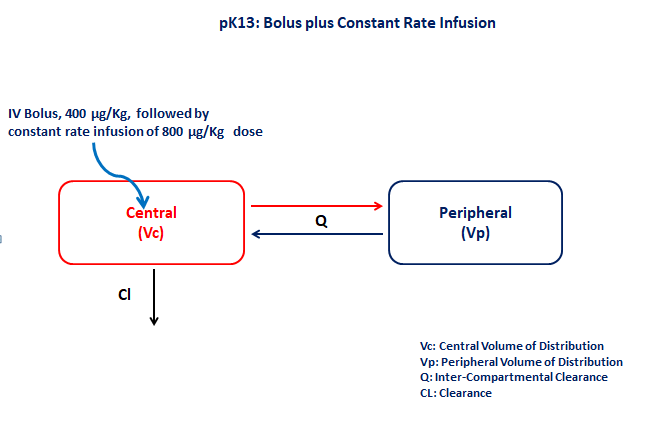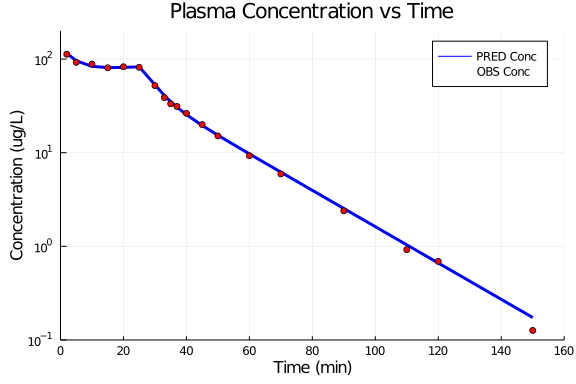# PK13 - Bolus plus constant rate infusion

### Background

• Structural model - Two compartment model with first order elimination

• Route of administration - IV-Bolus and IV-Infusion given simultaneously

• Dosage Regimen - 400 μg/kg IV-Bolus and 800 μg/kg IV-Infusion for 26 mins at time=0

• Number of Subjects - 1### Learning Outcome

• Write the differential equation for a two-compartment model in terms of Clearance and Volume

• Simulate data for both a bolus dose follwed by a constatnt rate infusion regimen

### Objective

The objective of this exercise is to fit data from a bolus follwed by a constant rate infusion using different model to provide a best fit to the data.

### Libraries

call the "necessary" libraries to get started

using Pumas
using Plots
using CSV
using StatsPlots
using Random


### Model

The given data follows a two compartment model in which the IV Bolus and IV-Infusion are administered at time=0

pk_13           = @model begin
@param begin
tvcl        ∈ RealDomain(lower=0)
tvvc        ∈ RealDomain(lower=0)
tvq         ∈ RealDomain(lower=0)
tvvp        ∈ RealDomain(lower=0)
Ω           ∈ PDiagDomain(4)
tvCMixRatio ∈ RealDomain(lower=0)
σ²_prop     ∈ RealDomain(lower=0)
end

@random begin
η           ~ MvNormal(Ω)
end

@pre begin
Cl          = tvcl * exp(η)
Vc          = tvvc * exp(η)
Q           = tvq * exp(η)
Vp          = tvvp * exp(η)
end

@dynamics begin
Central'    = -(Cl/Vc)*Central -(Q/Vc)*Central +(Q/Vp)*Peripheral
Peripheral' = (Q/Vc)*Central -(Q/Vp)*Peripheral
end

@derived begin
cp          = @. Central/Vc
dv          ~ @. Normal(cp, sqrt((cp*tvCMixRatio*σ²_prop)^2 + σ²_add^2))
end
end

PumasModel
Parameters: tvcl, tvvc, tvq, tvvp, Ω, tvCMixRatio, σ²_prop, σ²_add
Random effects: η
Covariates:
Dynamical variables: Central, Peripheral
Derived: cp, dv
Observed: cp, dv


### Parameters

• Cl - Clearance of central compartment (L/min/kg)

• Vc - Volume of central compartment (L/kg)

• Q - Inter-compartmental clearance (L/min/kg)

• Vp - Volume of peripheral compartment (L/kg)

• Ω - Between Subject Variability

• CMixRatio - Scaling factor

• σ - Residual Unexplained Variability

param = ( tvcl        = 0.344708,
tvvc        = 2.8946,
tvq         = 0.178392,
tvvp        = 2.18368,
Ω           = Diagonal([0.0, 0.0, 0.0, 0.0]),
tvCMixRatio = 1.00693,
σ²_prop     = 0.0571079,

(tvcl = 0.344708, tvvc = 2.8946, tvq = 0.178392, tvvp = 2.18368, Ω = [0.0 0
.0 0.0 0.0; 0.0 0.0 0.0 0.0; 0.0 0.0 0.0 0.0; 0.0 0.0 0.0 0.0], tvCMixRatio
= 1.00693, σ²_prop = 0.0571079, σ²_add = 0.1)


### Dosage Regimen

• Single dose of 400 μg/kg given as IV-Bolus at time=0

• Single dose of 800 μg/kg given as an IV-Infusion for 26 mins at time=0

ev1  = DosageRegimen(400, time=0, cmt=1)
ev2  = DosageRegimen(800, time=0, cmt=1, rate=30.769)
ev3  = DosageRegimen(ev1,ev2)
sub1 = Subject(id=1, events=ev3)

Subject
ID: 1
Events: 3


### Simulation

We will simulate the plasma concentration at the pre specified time points.

Random.seed!(123)
sim_sub1 = simobs(pk_13, sub1, param, obstimes=[2,5,10,15,20,25,30,33,35,37,40,45,50,60,70,90,110,120,150])


### DataFrame and Plot

Convert the simulation to a datframe and use the dataframe to make your necessary plots

df1 = DataFrame(sim_sub1)
@df df1 plot(:time, :cp, yaxis=:log,
title="Plasma Concentration vs Time", xlabel="Time (min)", ylabel="Concentration (ug/L)",
label= "PRED Conc", color=[:blue],linewidth=3,
xlims=(0,160), xticks=[0,20,40,60,80,100,120,140,160], ylims=(0.1,200))
@df df1 scatter!(:time, :dv, label="OBS Conc", color=[:red])